Created February 21, 2010
Last updated February 21, 2010
[ Japanese | English ]

### Object-oriented and UML

In this page , I will introduce the UML 1. And I will explain the element of the UML including the relation to the object-oriented design.
Recently , the UML is come into question at the Japan Information-Technology Engineers Examination. Therefore, if you want to pass the examination, study it.
If you think to pass the OCUP or UMTP, you don't need to read this page , because I think that you already know.

-----
Note.
1: In this page , the UML version is 2.0.

#### 1. What is the UML?

The UML (Unified Modeling Language) is a language used at system analysis and design.
As for a language , you may think difficalt like Japanese or programming language. But the UML is not so. You may think the way of drawing diagrams.
Since the UML is an ordinary language , you can use free. ( You must use in the object-oriented design. You must not use in the Waterfall model... No! It is not so 1. You can use free. )
The merit of the UML:
1. The name and means of the element is defined by the UML specification 2.
This is very important when you communicate other person.
There is a merit that you don't need to draw explanatory notes too 3.
2. It is a diagram base.
I think that a diagram is more understandable than a letter.
I think that there is a merit of the visualization too.

Of course , the UML is not complete.
1. The UML can not express all.
For example , It is difficult for you to draw screen transition chart by the UML.
The use case diagram is able to express the function of the system , but not able to express the non-functional requirement 4.
In the UML , you can expand. But , still , there are unexpressible things 5.
If the UML is able , there is also a case that the tool of the UML don't support.
2. There is a possibility that the retrieval of the word (attribute name etc.) is not easily.
This problem is not by the UML but by the tools. For example ,can you do the Grep search from the UML document ?
( Sooner or later , I think that you can do. However, can you do now? )
3. There is a possibility that the recycling drawn diagrams is difficult.
Wnen you draw diagrams by a tool , can you recycle the diagram by other tool?
( Sooner or later , I think that you can do. However, can you do now? )

I want to explain others, but because it becomes long , I will end the explanation of the UML. If you want to know the more , please , search by the Google or Books.

-----
Note.
1: The generalized expression:
The UML is usable is not dependent by the analysis and design approach like the POA , DOA and OOA , and is not dependent by the development process like the waterfall model , prototype model and spiral model.

Because the UML is often explained with the object-oriented, I want you to pay attention.
2: There is a case that the name and so on of the element differ from the UML version.
Therefore , when you draw an UML diagram , you should write the UML version.
3: This is not correct. Because you can extend the UML ( you can define a stereotype and so on. ). In this case , the explanatory notes is needed.
And , when you communicate the person who don't know the UML , the explanatory notes of the UML is needed.
4: For example , when I design in Excel VBA , I think that most important non-functional requirement is speed. Of course , there are more.
5: For example , you can not express in, out and inout at the operation of the class in a class diagram.
This(in , out and inout) is defined in the UML ( Fig 7.10 ). But I don't know the expression.

#### 2. What is an use case diagram?

The "use case diagram" is a diagram that expresses the relation to existing system , user and function of the system that you will create. (Of course , you may use by other uses.)
The use case diagram is not related to object-oriented design. ( Note. But related to object-oriented analysis. )
Because , there is a case that when you draw an use case diagram and think the realization , you may stop the object-oriented design.

Figure 1 is the example of the use case diagram. This is a system of the books control ( control of place and number of books ) 1.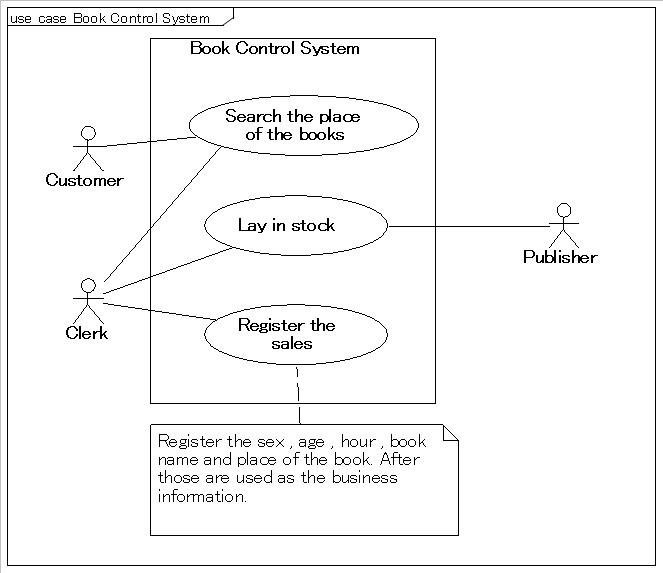Figure 1 - The example of the use case diagram (When the image is clicked, what expands to another screen is displayed.)
This is a system of the books control ( control of place and number of books ) 1.

-----
Note.
1: This example is for the explanation. Therefore , you can not use by the actual development.
For example , in the actual development , you may consider the cost of books, but it is omitted in Figure 1.
After that, the use case ( oval in Figure 1 ) must be made the same size of the means , but it may not be so.

#### 3. What is an activity diagram?

Chiefly the "activity diagram" expresses the process flow and business flow.
I think that it is like the flow chart.

I think that it is not related to object-oriented design. There is a case that it is used when you want express the process flow of the operation of the class. But , this is not object-oriented design but structured programming 1.

Figure 2 is the example of the activity diagram. This is the business flow that the customer buy the book ( go to the cashier and pay ) 2.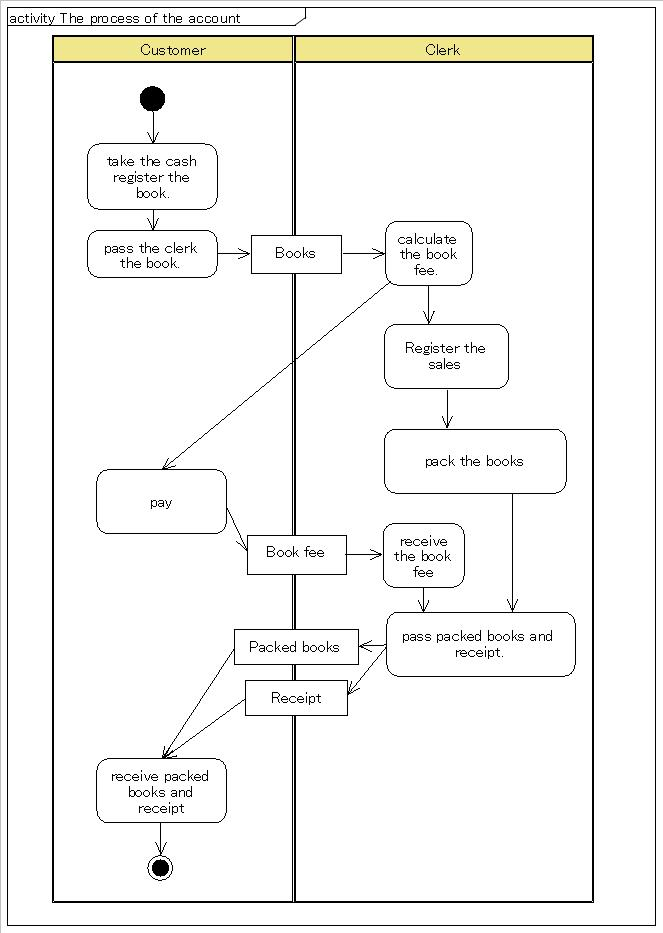Figure 2 - The example of the activity diagram (When the image is clicked, what expands to another screen is displayed.)
The business flow that the customer buy the book ( go to the cashier and pay ) 2

-----
Note.
1: Even if you design in object-oriented , the design of the operation is the same as structured programming.
2: This example is also for the explanation. Therefore , you can not use by the actual development.

#### 4. What is a class diagram?

The "class diagram" is a diagram that expresses the attributes and operations of the classes and associations of the classes.

I think that the class diagram is related to object-oriented design. When we design in object-oriented , we often draw the class diagram. Of course , there are not things that we must draw it and we can draw it by other use. 1

Figure 3 is the example of the class diagram. This is the class diagram that express the data structure needed by the books control.2 , 3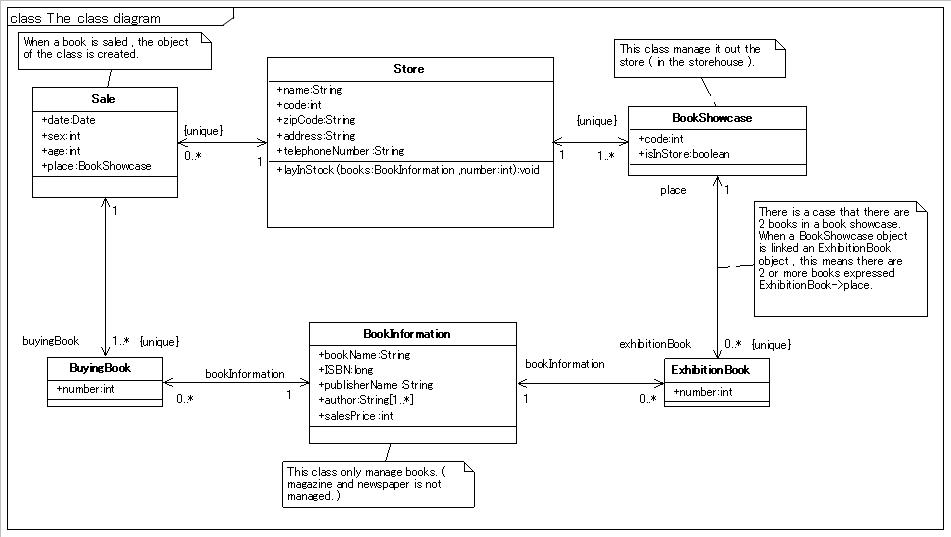Figure 3 - The example of the class diagram (When the image is clicked, what expands to another screen is displayed.)
This is the class diagram that express the data structure needed by the books control.2 , 3

-----
Note.
1: For example , instead of the ER diagram , you can draw the class diagram.
2: This example is also for the explanation. Therefore , you can not use by the actual development.
3: I think that the class diagram is the most difficult among the UML diagrams.

#### 5. What is a sequence diagram?

The "sequence diagram" is a diagram that expresses the call of the operation between objects by the time series. 1
Chiefly , it is used when we want to express the process flow.

Figure 4 is the example of the sequence diagram. This is a sequence diagram of the case that customer search the book by the books control system.2 , 3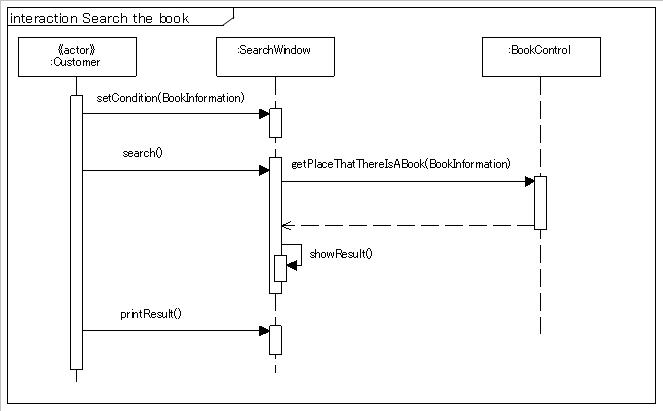Figure 4 - The example of the sequence diagram (When the image is clicked, what expands to another screen is displayed.)
This is a sequence diagram of the case that customer search the book by the books control system.2 , 3

-----
Note.
1: If there are not asynchronous calls , the flow is from top to bottom.
If there are asynchronous calls ... , please search by yourself. ( * it is a range of the OCUP Fundamental. )
2: This example is also for the explanation. Therefore , you can not use by the actual development.
3: In general , the actor is expressed the stick man ( this is used in the use case diagram ). But , because tool is unsupported , I use the "actor" keyword.

#### 6. What is a state machine diagram?

The "state machine diagram" 1 is a diagram that expresses the state transition.
Chiefly , this is used when we want to express the state transition of the object , system and so on.

Figure 5 is the example of the state machine diagram. This is the diagram that express the state of the bookstore ( opened and closed )2.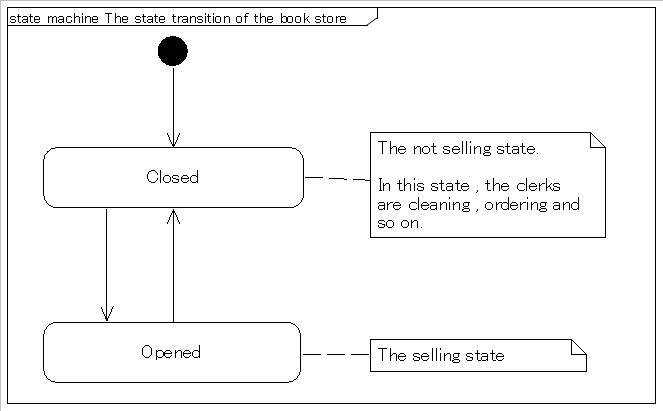Figure 5 - The example of the state machine diagram (When the image is clicked, what expands to another screen is displayed.)
The diagram that express the state of the bookstore ( opened and closed )2

-----
Note.
1: In UML 1.5 , this is called "state chart diagram".
2: This example is also for the explanation. Therefore , you can not use by the actual development.

#### 7. The common used elements of the UML

The "comment" 1, "frame" , "stereotype" 2 is not a diagram , but is often used. Therefor , I think that you should know.

1. Comment
This is used when we want to write comments and limiting condition.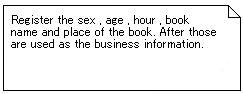Figure 6 - The example of the comment (When the image is clicked, what expands to another screen is displayed.)

2. Frame
This expresses the kind , name and territory of the diagram.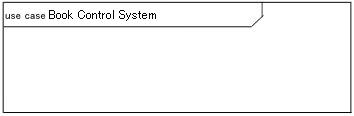Figure 7 - The example of the frame (When the image is clicked, what expands to another screen is displayed.)

3. Stereotype
This expresses that the element 3 in the UML diagram is special. Because the notation is the same as the "keyword" 4 , we often mistake.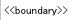Figure 8 - The example of the stereotype

-----
Note.
1: In UML 1.5 , this is called "Note".
2: The list of the standard stereotype is written on the page 669 (Annex C Standard Stereotypes) in the UML Specification 2.0.
3: The "element" is thing that composes the class , object , state and so on in UML.
4: The list of the keyword is written on the page 663 (Annex B UML Keywords) in the UML Specification 2.0.

#### 8. Other diagram

I introduced the use case diagram , activity diagram , class diagram , sequence diagram and state machine diagram. And there are other diagrams in UML.But , I think that you are not having difficulty.

#### 9. Reference

The following tables are pages that I referred to create this page.

 No. Linked Website Name Description Linked date 1 OMG Document -- UML Superstructure Specification, v2.0 February 21, 2010 2 Structured programming - Wikipedia, the free encyclopedia This page exlain the structureed programming. February 21, 2010 3 Unified Modeling Language - Wikipedia, the free encyclopedia This page exlain the UML. February 21, 2010